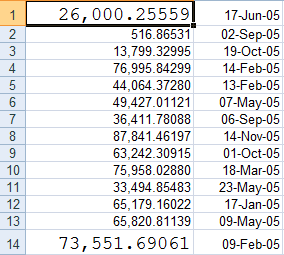﻿ Resize Columns in C1Excel# Resize Columns in C1Excel

C1XLBook can be used to load existing Excel files or create new ones. It may also be used for adding sheets, styles, hyperlinks, images, headers and footers, page breaks etc. If a cell is assigned a style that makes the cell-text's width larger than the cell width, i.e. the Column's width, we get a distorted display. This is true for MS-excel as well. The fix is simple - resize the column accordingly. However, C1XLBook doesn't have an inbuilt method/property, as yet, to implement this. So we put in a bit of code to make it happen. Lets begin by placing a c1XLBook component (c1XLBook1) to the windows Form. Then create an excel sheet with two columns. Also, create three styles (s1,s2,s3) and assign a large Font to one of the styles (s2) to make sure that the cell text gets wider than the column's width.

``````
c1XLBook1.Clear();
/\*Create test Styles\*/
XLStyle s1 = new XLStyle(c1XLBook1);
XLStyle s2 = new XLStyle(c1XLBook1);
XLStyle s3 = new XLStyle(c1XLBook1);
s1.Format = "#,##0.00000";
s2.Format = "#,##0.00000";
/\*Assign a large enough Font\*/
s2.Font = new Font("Courier New", 14);
s3.Format = "dd-MMM-yy";

C1.C1Excel.XLSheet sheet = c1XLBook1.Sheets;
Random r = new Random();
for (int i = 0; i < 100; i++)
{
sheet[ i, 0 ].Value = r.NextDouble() * 100000;
/\*Assign the style with enlarged Font to every cell in 13th Row of first Column\*/
sheet[ i, 0 ].Style = (i % 13 == 0) ? s2 : s1;
}
for (int i = 0; i < 100; i++)
{
sheet[ i, 1 ].Value = new DateTime(2005, r.Next(1, 12), r.Next(1, 28));
sheet[ i, 1 ].Style = s3;
}

string tempdir = Application.ExecutablePath.Substring(0,
Application.ExecutablePath.LastIndexOf("\\\") + 1);

/\*Save sheet with default column width\*/
c1XLBook1.Save(tempdir + @"defaultWidth.xls");

``````

However, this excel sheet is the one with default column width - no resizing at all. Here's how we can resize the columns.

``````
private void AutoSizeColumns(XLSheet sheet)
{
/\*No Graphics instance available because there's no Paint event\*/
/\*Create a Graphics object using a handle to current window instead\*/
using (Graphics g = Graphics.FromHwnd(IntPtr.Zero))
{
/\*Traverse rows and columns\*/
for (int c = 0; c < sheet.Columns.Count; c++)
{
int colWidth = -1;
for (int r = 0; r < sheet.Rows.Count; r++)
{
/\*Get cell value\*/
object value = sheet[r, c].Value;
if (value != null)
{
string text = value.ToString();

/\*Get Style for this cell\*/
C1.C1Excel.XLStyle s = sheet[r, c].Style;
if (s != null && s.Format.Length > 0 && value is IFormattable)
{
string fmt = XLStyle.FormatXLToDotNet(s.Format);
/\*get formatted text\*/
text = ((IFormattable)value).ToString(fmt, CultureInfo.CurrentCulture);
}

Font font = this.c1XLBook1.DefaultFont;
if (s != null && s.Font != null)
{
font = s.Font;
}

/\*Get size of drawn string according to its Font\*/
Size sz = Size.Ceiling(g.MeasureString(text + "XX", font));

if (sz.Width > colWidth)
colWidth = sz.Width;
}
}
/\*Set columns width\*/
if (colWidth > -1)
sheet.Columns[c].Width = C1XLBook.PixelsToTwips(colWidth);
}
}
}

``````

Now, simply call this method, with the excel sheet that we designed, passed as parameter.

``````
/\*Resize columns\*/
AutoSizeColumns(sheet);

/\*Save sheet with resized columns\*/
c1XLBook1.Save(tempdir + @"autoSized.xls");

System.Diagnostics.Process.Start(tempdir + @"autoSized.xls");

``````

And then this is how the resized column looks like.However, the code doesn't take into account merging or wrapping of cells. If you have code that implements this and wish to share; please free to post it here :) Refer to the samples below for complete implementation. Download CS sample Download VB SampleGrapeCity

GrapeCity Developer Tools